Get a timely done, PLAGIARISM-FREE paper
from our highly-qualified writers!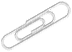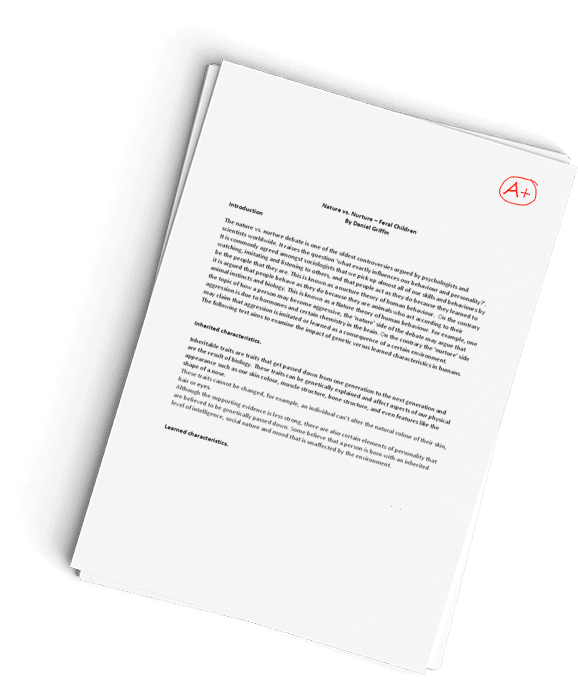## FINAL LAB PRACTICAL–General Chemistry II Lab

Part I: Equilibrium Constant Determination:

The equilibrium for the formation of FeSCN2+ has been achieved by combining 0.002 M solutions of Fe(NO3)3 and NaSCN.

Reaction studied:      Fe3+  +  SCN1-  <=>  FeSCN2+, Kf = [FeSCN2+]/[Fe3+][ SCN1-]

Based on Beer’s Law concept, we achieved a linear plot by various dilutions of reactants (these data are not given). The linear equation is shown below.

A  = 2622.52 x [FeSCN2+] -2.691E-4           R =  0.99965

Remember to use the formula CiVi = CfVf to determine the initial concentrations right before they react. (Note: Vf is 6.00 mL every time.)

Table 1: Initial concentration of Fe3+ and SCN solutions.                           (10 points)

 Soln # Volume of 2.00E-3 M Fe3+ (mL) Volume of 2.00E-3 M SCN1- (mL) Volume of water (mL) Total Volume (mL) [Fe3+]o M [SCN1-]o M Blank 3.00 0.00 3.00 6.00 1.00E-3 0 1B 1.00 3.00 2.00 6.00 3.33E-4 1.00E-3 2B 2.00 3.00 1.00 6.00 6.67E-4 1.00E-3 3B 3.00 3.00 0.00 6.00 1.00E-3 1.00E-3 4B 3.00 2.00 1.00 6.00 1.00E-3 6.67E-4 5B 3.00 1.00 2.00 6.00 1.00E-3 3.33E-4

Use Beer’s Law to determine the concentration from the absorbance provided and use ICE charts to determine the equilibrium concentrations and the Kf.

(15 points)

 Soln # Absorbance measured [FeSCN2+]eq M [Fe3+]eq M [SCN1-]eq M Kf Blank — — — — — 1B 0.105 0.0000399 0.0002901 0.0009601 14.33 2B .225 0.0000857 0.0005813 0.0009143 161.25 3B .345 0.000131 0.000869 0.000869 173.47 4B .451 0.000172 0.000828 0.000495 419.63 5B .564 0.000215 0.000785 0.000118 2321.06

Calculate the average Kf and its standard deviation and perform the 4D test, if necessary, i.e., based on the discarding of n unwanted value.             (5 points)

### STANDARD DEVIATION

Mean = 14.33+161.25+173.47+419.63+2321.06 =3089.74/5 =617.95

 x x-mean (x-mean)2 14.33 -603.62 364357.1044 161.25 -456 207936 173.47 -444.48 197562.47 419.63 -198.32 39330.8224 2321.06 1703.11 2900583.672 Sum=3089.74 0 3709770.069

Variance = 3709770.069/ 5-1 = 741954.0138

Standard deviation =

= 861.4

(a) Show calculations for the initial diluted concentrations of Fe3+ and SCN–

(5 points)

 [Fe3+]o M [SCN1-]o M 1B = CiVi = CfVf                                                                                                                             2.00E-3 M X 1.00 = 6.00 X Cf                                   Cf   = 3.333 E-4 1B = CiVi = CfVf                                                                                                                              2.00E-3 M X 3.00 = 6.00 X Cf                                   Cf   = 1.00 E-3 2B = CiVi = CfVf                                                                                                                             2.00E-3 M X 2.00 = 6.00 X Cf                                   Cf   = 6.67E-4 2B = CiVi = CfVf                                                                                                                             2.00E-3 M X 3.00 = 6.00 X Cf                                   Cf   = 1.00 E-3 3B = CiVi = CfVf                                                                                                                              2.00E-3 M X 3.00 = 6.00 X Cf                                   Cf   = 1.00 E-3 3B = CiVi = CfVf                                                                                                                              2.00E-3 M X 3.00 = 6.00 X Cf                                   Cf   = 1.00 E-3 4B = CiVi = CfVf                                                                                                                             2.00E-3 M X 3.00 = 6.00 X Cf                                   Cf   = 1.00 E-3 4B = CiVi = CfVf                                                                                                                              2.00E-3 M X 2.00 = 6.00 X Cf                                   Cf   = 6.67 E-4 5B = CiVi = CfVf                                                                                                                             2.00E-3 M X 3.00 = 6.00 X Cf                                   Cf   = 1.00 E-3 5B = CiVi = CfVf                                                                                                                              2.00E-3 M X 1.00 = 6.00 X Cf                                   Cf   = 3.333 E-4

(b) Show the determination of equilibrium FeSCN2+ concentration from absorbance using Beer’s Law linear regression equation.                                            (5 points)

1B

A = 2622.52 x [FeSCN2+] -2.691E-4

0.105 = 2622.52 x [FeSCN2+] -2.691E-4

0.105 – 0.0002691 = 2622.52[FeSCN2+]

[FeSCN2+] =  = 3.99 E-5

2B

A = 2622.52 x [FeSCN2+] -2.691E-4

0.225 = 2622.52 x [FeSCN2+] -2.691E-4

0.225 – 0.0002691 = 2622.52[FeSCN2+]

[FeSCN2+] =  = 8.57E-5

3B

A = 2622.52 x [FeSCN2+] -2.691E-4

0.345 = 2622.52 x [FeSCN2+] -2.691E-4

0.345 – 0.0002691 = 2622.52[FeSCN2+]

[FeSCN2+] =  = 1.31E-4

4B

A = 2622.52 x [FeSCN2+] -2.691E-4

0.451 = 2622.52 x [FeSCN2+] -2.691E-4

0.451 – 0.0002691 = 2622.52[FeSCN2+]

[FeSCN2+] =  = 1.72E-4

5B

A = 2622.52 x [FeSCN2+] -2.691E-4

0.564= 2622.52 x [FeSCN2+] -2.691E-4

0.564 – 0.0002691 = 2622.52[FeSCN2+]

[FeSCN2+] =  = 2.15E-4

(c) Show the ce table to calculate the equilibrium concentrations of Fe3+ and SCN and Kf calculation.                                                                                                     (10 points)

From, Fe3+  +  SCN1-  <=>  FeSCN2+

Trial 1B

Fe3+                            SCN1                                      FeSCN2+

 Initial 0.00033 0.001 0 Change 0.00033-0.0000399 0.001-0.0000399 3.99e-05 Equilibrium 0.0002901 0.0009601 3.99e-05

Kf = [FeSCN2+]/[Fe3+][ SCN1-]   = (0.0000399)/ (0.002901)X (0.0009601)

Kf = 14.33

Trial 2B

Fe3+                            SCN1                                      FeSCN2+

 Initial 0.000667 0.001 0 Change 0.000667-0.0000857 0.001-0.0000857 8.57e-05 Equilibrium 0.0005813 0.0009143 8.57e-05

Kf = [FeSCN2+]/[Fe3+][ SCN1-]   = (0.0000857)/ (0.0005813)X (0.0009143)

Kf = 161.25

Trial 3B

Fe3+                            SCN1                                      FeSCN2+

 Initial 0.001 0.001 0 Change 0.001-0.000131 0.001-0.000131 0.000131 Equilibrium 0.000869 0.000869 0.000131

Kf = [FeSCN2+]/[Fe3+][ SCN1-]   = (0.000131)/ (0.000869)X (0.000869)

Kf = 173.47

Trial 4B

Fe3+                            SCN1                                      FeSCN2+

 Initial 0.001 0.000667 0 Change 0.001-0.000172 0.000667-0.000172 0.000172 Equilibrium 0.000828 0.000495 0.000172

Kf = [FeSCN2+]/[Fe3+][ SCN1-]   = (0.000172)/ (0.000495)X (0.000828)

Kf = 419.66

Trial 5B

Fe3+                            SCN1                                      FeSCN2+

 Initial 0.001 0.000333 0 Change 0.001-0.000215 0.000333-0.000215 0.000215 Equilibrium 0.000785 0.000118 0.000215

Kf = [FeSCN2+]/[Fe3+][ SCN1-]   = (0.000215)/ (0.000785)X (0.000118)

Kf = 2321.06

(d) Write a summary of the argument on the formation of FeSCN2+ complex (10 points)

The deductions from the experiments above indicate that absorbance is directly proportional to the concentration of the reagents. Trials 2B and 3B have close results. The Kf value for ttrials1B and 5B differs from others by a big margin. These are the unwanted values since they are out of range, even if you consider the standard deviation. Therefore, trials 2 and 3 give a clear hypothesis to illustrate the relationship between the Beers regression law and how they relate to absorbance and concentrations of different solutions.

(II) Standard Cell Potentials (Voltaic Cells):

Galvanic Cell

A galvanic cell is an important electrochemical cell. It is named after Luigi Galvani, an Italian physicist. It is also called a Voltaic cell, after an Italian physicist, Alessandro Volta. A galvanic cell generally consists of two different metal rods called electrodes. Each electrode is immersed in a solution containing its onions, and these form a half-cell. Each half-cell is connected by a salt bridge or separated by a porous membrane. The solutions in which the electrodes are immersed are called electrolytes.

The chemical reaction that takes place in a galvanic cell is the redox reaction. One electrode acts as the anode in which oxidation takes place, and the other acts as the cathode in which reduction takes place.  The best example of a galvanic cell is the Daniell cell.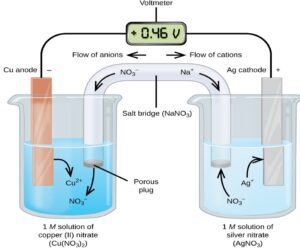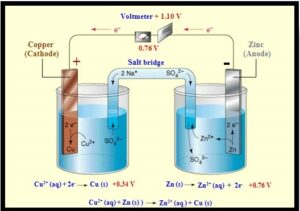The cell voltage is proportional to ΔG, the change in Gibbs free energy. The ΔG of the reaction could be harnessed to do useful electrical work. This thermodynamic equation gives the value of ΔG.

ΔG = ΔG° + RT ln Q  — (1)

The relationship between cell voltage, E, and ΔG for the cell reaction is given by the following equation.

DG = -nFE, or E = -DG/nF —(2), where n is number of electrons, F = 96485 J/mol.V

The Nernst equation showing the cell potential can be finally written as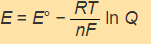(or)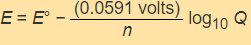Where E° = E°1/2(reduction) − E°1/2(oxidation)

E° = E°1/2(cathode) − E°1/2(anode)

In this investigation, you will make the following voltaic cells by using the following simulation:

http://amrita.olabs.edu.in/?sub=73&brch=8&sim=153&cnt=4

Activity series: Use cell notation and perform the virtual lab.

 Part I Part II Anode             (oxidation) Cathode       (reduction) Anode            (oxidation) Cathode       (reduction) (1)   Mg/Mg2+(0.05 M) || Zn2+ (0.1 M)/Zn  (2)   Mg/Mg2+( (0.05 M) || 2Ag+ (0.1 M)/2Ag       (3)   Mg/Mg2+( (0.05 M) || Cu2+(0.1 M)/Cu (4)   Mg/Mg2+( (0.05 M) || Fe2+(0.1 M)/Fe (5)   Mg/Mg2+(0.05 M) || Ba2+ (0.1 M)/Ba (6)   Zn/Zn2+(0.05 M) || Ba2+ (0.1 M)/Ba           (7)   Zn/Zn2+(0.05 M) || Mg2+ (0.1 M)/Mg             (8)   Zn/Zn2+ (0.05 M) || 2Ag+ (0.1 M)/2Ag  (9)   Zn/Zn2+ (0.05 M) || Cu2+(0.1 M)/Cu           (10)   Zn/Zn2+ (0.05 M) || Fe2+(0.1 M)/Fe

Procedure:

You will create a series of voltaic cell models in two different parts: (i) Mg involves oxidation consistently, and other ions involve reduction, and (ii) Zn involves oxidation consistently, and other ions involve reduction.

Set the concentration of solution in the anode part (left) as 0.05 M, whereas the concentration of the solution in the cathode part is 0.1 M.

This setup is consistent for all 10 cell models where you can see the type of solutions (e.g., aqueous BaCl2, MgCl2, AgNO3, ZnSO4, CuSO4 and FeSO4) where cations are ionized in the solution.

Part I:

• Select the electrode Mg in the anode part (top), where MgCl2 (the corresponding ionic solution) will appear in the beaker by default.
• Select the electrode Zn in the cathode part (bottom), where ZnSO4 (the corresponding ionic solution) will appear in the beaker by default. This is model #1.
• Observe the cell potential (Ecell) from the digital voltmeter.
• Repeat the same procedure with the anode (Mg) and various cathodes (Ag, Cu, Fe and Ba) to complete the rest of the models (Models 2-5).

Part II:

• Select the electrode Zn in the anode part (top), where ZnSO4 (the corresponding ionic solution) will appear in the beaker by default.
• Select the electrode Ba in the cathode part (bottom), where BaCl2 (the corresponding ionic solution) will appear in the beaker by default. This is model #6
• Observe the cell potential (Ecell) from the digital voltmeter.
• Repeat the same procedure with the anode (Zn) and various cathodes (Ag, Cu, Fe and Ba) to complete the rest of the models (Models 7-10).

Table: Cell potential measured for various voltaic cells (by using simulation) in comparison with the theoretical values.                     (10 points)

 Voltaic cell Electrodes Anode Cathode Ecell, V (Exp.) Ecell, V (Pred.)a % Error 1 Mg & Zn Mg/Mg2+(0.05 M) Zn2+ (0.1 M)/Zn 1.619 1.619 0 2 Mg & Ag Mg/Mg2+( (0.05 M) 2Ag+ (0.1M)/2Ag 3.17 3.25 -2.52 3 Mg & Cu Mg/Mg2+( (0.05 M) Cu2+(0.1 M)/Cu 2.71 2.79 -2.95 4 Mg & Fe Mg/Mg2+( (0.05 M) Fe2+(0.1 M)/Fe 1.93 2.01 -4.14 5 Mg & Ba Mg/Mg2+(0.05 M) Ba2+ (0.1 M)/Ba -0.53 -0.44 -2.00 6 Zn & Ba Zn/Zn2+(0.05 M) Ba2+ (0.1 M)/Ba -2.14 -2.05 16.9 7 Zn & Mg Zn/Zn2+(0.05 M) Mg2+ (0.1 M)/Mg -1.61 -1.52 5.59 8 Zn & Ag Zn/Zn2+ (0.05 M) 2Ag+ (0.1 M)/2Ag 1.56 1.64 -5.12 9 Zn & Cu Zn/Zn2+ (0.05 M) Cu2+(0.1 M)/Cu 1.1 1.18 -7.27 10 Zn & Fe Zn/Zn2+ (0.05 M) Fe2+(0.1 M)/Fe 0.32 0.40 25.0

a Finish the answering question (a) and enter values here.

(a) Calculate the predicted Ecell value for each cell by the Nernst Equation. (20 points)

where n = 2 and Q = [0.05]/[0.1] à both are consistent in all 10 models

(1) Model calculation for Model #1: Collect the reduction potential from the table at the bottom of the PPT or other resources.

Eo = Eo(cathode) – Eo(anode) = (-0.76 V) – (-2.37 V) = +1.61 V

E = Eo – (0.0591/n) (log Q) = 1.61 – (0.0591/2) (log [0.05/0.1]) = 1.619 V

 From the Desmos scientific calculator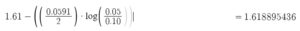From the Virtual Lab: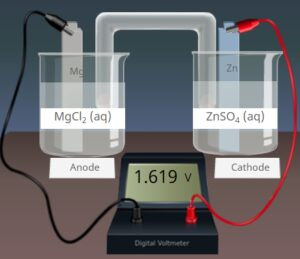### The calculation for Model #2:

Eo = Eo(cathode) – Eo(anode) = (+0.80V) – (-2.37 V) = +3.17 V

E = Eo – (0.0591/n) (log Q) = 3.17 – (0.0591/2) (log [0.05/0.1]) = 3.25 V

Calculation for Model #3:

Eo = Eo(cathode) – Eo(anode) = (+0.34V) – (-2.37 V) = +2.71 V

E = Eo – (0.0591/n) (log Q) = 2.71 – (0.0591/2) (log [0.05/0.1]) = 2.79 V

### The calculation for Model #4:

Eo = Eo(cathode) – Eo(anode) = (-0.44V) – (-2.37 V) = +1.93 V

E = Eo – (0.0591/n) (log Q) = 1.93 – (0.0591/2) (log [0.05/0.1]) = 2.01V

### The calculation for Model #5:

Eo = Eo(cathode) – Eo(anode) = (-2.90V) – (-2.37 V) = -0.53 V

E = Eo – (0.0591/n) (log Q) = -0.53 – (0.0591/2) (log [0.05/0.1]) = -0.44V

### Calculation for Model #6:

Eo = Eo(cathode) – Eo(anode) = (-2.90V) – (-0.76 V) = -2.14 V

E = Eo – (0.0591/n) (log Q) = -2.14 – (0.0591/2) (log [0.05/0.1]) = -2.05 V

### Calculation for Model #7:

Eo = Eo(cathode) – Eo(anode) = (-2.37V) – (-0.76 V) = -1.61 V

E = Eo – (0.0591/n) (log Q) = -1.61 – (0.0591/2) (log [0.05/0.1]) = -1.52 V

### Calculation for Model #8:

Eo = Eo(cathode) – Eo(anode) = (+0.80V) – (-0.76 V) = +1.56 V

E = Eo – (0.0591/n) (log Q) = 1.56 – (0.0591/2) (log [0.05/0.1]) = 1.64V

### Calculation for Model #9:

Eo = Eo(cathode) – Eo(anode) = (+0.34V) – (-0.76 V) = +1.1 V

E = Eo – (0.0591/n) (log Q) = 1.1 – (0.0591/2) (log [0.05/0.1]) = 1.18V

### Calculation for Model #10:

Eo = Eo(cathode) – Eo(anode) = (-0.44V) – (-0.76 V) = +0.32 V

E = Eo – (0.0591/n) (log Q) = 0.32 – (0.0591/2) (log [0.05/0.1]) = 0.40V

(b) Write an overall redox reaction for each voltaic cell first and then half-reactions, which are labeled “oxidation” and “reduction.”      (10 points)

 Model 1: Mg/Mg2+ || Zn2+/Zn   Redox: Mg(s) + Zn2+(aq) à Mg2+(aq) + Zn(s)   Oxidation: Mg à Mg 2+ + 2e– Reduction: Zn2+ + 2e–  àZn Model 6:     Zn/Zn2+ || Ba2+/Ba   Oxidation: Zn à Zn 2+ + 2e–   Reduction: Ba2+ + 2e–  àBa Model 2:     Mg/Mg2+ || 2Ag+/ 2Ag   Oxidation: Mg à Mg 2+ + 2e–   Reduction: 2Ag2+ + 2e–  à2Ag Model 7:   Zn/Zn2+ || Mg2+/Mg   Oxidation: Zn à Zn 2+ + 2e–   Reduction: Mg2+ + 2e–  àMg Model 3:   Mg/Mg2+ || Cu2+/Cu     Oxidation: Mg à Mg 2+ + 2e–   Reduction: Cu2+ + 2e–  àCu Model 8: Zn/Zn2+ || 2Ag+/2Ag   Oxidation: Zn à Zn 2+ + 2e–   Reduction: 2Ag2+ + 2e–  à2Ag Model 4: Mg/Mg2+ || Fe2+/Fe   Oxidation: Mg à Mg 2+ + 2e–   Reduction: Fe2+ + 2e–  àFe Model 9: Zn/Zn2+ || Cu2+/Cu   Oxidation: Zn à Zn 2+ + 2e–   Reduction: Cu2+ + 2e–  àCu Model 5: Mg/Mg2+ || Ba2+/Ba   Oxidation: Mg à Mg 2+ + 2e–   Reduction: Ba2+ + 2e–  àBa Model 10: Zn/Zn2+ || Fe2+/Fe   Oxidation: Zn à Zn 2+ + 2e–   Reduction: Fe2+ + 2e–  àFe

(c) Write a summary of the argument based on this simulation lab. (10 points)

From the simulations, it is evident that the standard electrode potentials provided determine whether a reaction will take place or not.  Also, the most reactive metals from the table undergo oxidation, thus strong reducing agents. The least reactive metals undergo reduction, hence strong oxidizing agents. The value of the electrode potential determines the position of a metal in a reactivity series. For example, copper has an electrode potential of +0.34 V, while magnesium has -2.37V. Therefore, copper cannot displace magnesium from a solution of its ions, but magnesium can displace copper. Overall, negative electrode potential from a complete cell indicates that a reaction cannot take place and vice versa.

Appendix Table: Reduction potential for various electrode and reduction reactions.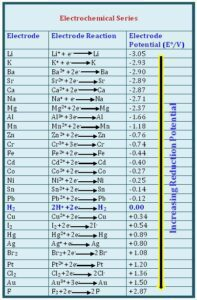ORDER A PLAGIARISM-FREE PAPER HERE

We’ll write everything from scratch

Question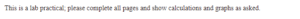FINAL LAB PRACTICAL–General Chemistry II Lab

This is a lab practical; please complete all pages and show calculations and graphs as asked.

Have a similar assignment? "Place an order for your assignment and have exceptional work written by our team of experts, guaranteeing you A results."## Our Service Charter

1. Professional & Expert Writers: Eminence Papers only hires the best. Our writers are specially selected and recruited, after which they undergo further training to perfect their skills for specialization purposes. Moreover, our writers are holders of masters and Ph.D. degrees. They have impressive academic records, besides being native English speakers.

2. Top Quality Papers: Our customers are always guaranteed of papers that exceed their expectations. All our writers have +5 years of experience. This implies that all papers are written by individuals who are experts in their fields. In addition, the quality team reviews all the papers before sending them to the customers.

3. Plagiarism-Free Papers: All papers provided by Eminence Papers are written from scratch. Appropriate referencing and citation of key information are followed. Plagiarism checkers are used by the Quality assurance team and our editors just to double-check that there are no instances of plagiarism.

4. Timely Delivery: Time wasted is equivalent to a failed dedication and commitment. Eminence Papers are known for the timely delivery of any pending customer orders. Customers are well informed of the progress of their papers to ensure they keep track of what the writer is providing before the final draft is sent for grading.

5. Affordable Prices: Our prices are fairly structured to fit in all groups. Any customer willing to place their assignments with us can do so at very affordable prices. In addition, our customers enjoy regular discounts and bonuses.

6. 24/7 Customer Support: At Eminence Papers, we have put in place a team of experts who answer all customer inquiries promptly. The best part is the ever-availability of the team. Customers can make inquiries anytime.